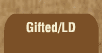Mathematics and Dyslexia

Tips for Learning the Times Tables

Tools for the Times Tables & Workbook

Home > Math > times tables practice > fours times tables (mixed)

## The Fours Times Tables

These may be the first ones that you really need to practice. If you don't do as well here as you've done with the earlier stages, spend some more time with these. It's worth it.

Don't move on until these are fast and easy. If you think you know them, go back to the fives, then come back to these and see if you do these as quickly. If not, practice them some more and try it again. If two or three of them give you a lot of trouble -- 4 x 6 and 7 x 4 are famous for being harder -- just practice them, then mix them back in with the rest of them.

Numbers multiplied by four are always even. Multiplying by four is the same thing as multiplying by two -- twice. So, if you double something, then double it again, you're multiplying by four.

So, you can figure out that 4 x 3 is twelve by doubling 3 to get 6... then doubling 6 to get 12.

OR... practice this page until you just know them... so when someone says 4 x 3, you think "12."

1. 8 x 4=
2. 4 x 1=
3. 4 x 7=
4. 0 x 4=
5. 10 x 4=
6. 4 x 2=
7. 4 x 4=
8. 9 x 4=
9. 4 x 3=
10. 4 x 6=
11. 4 x 5=
12. 6 x 4=
13. 4 x 7=
14. 8 x 4=
15. 4 x 9=